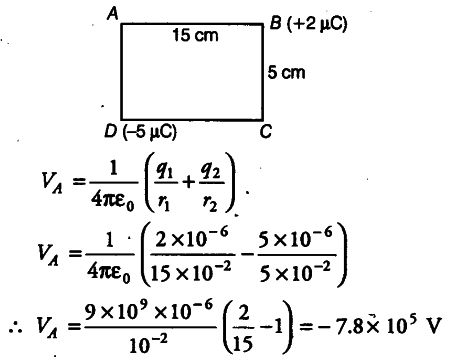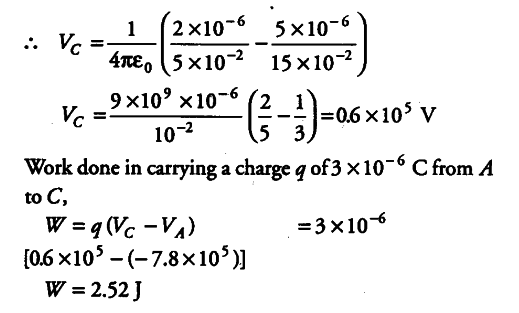# The sides of a rectangle ABCD are 15 cm and 5 cm as shown in the figure

The sides of a rectangle ABCD are 15 cm and 5 cm as shown in the figure. Point charges of -5 µC, +2 µC are placed at the comers D and B, respectively. Calculate electric potential at A and C. Also, calculate work done in carrying a charge of 3 µC from A to C.

As, it is clear in figure,